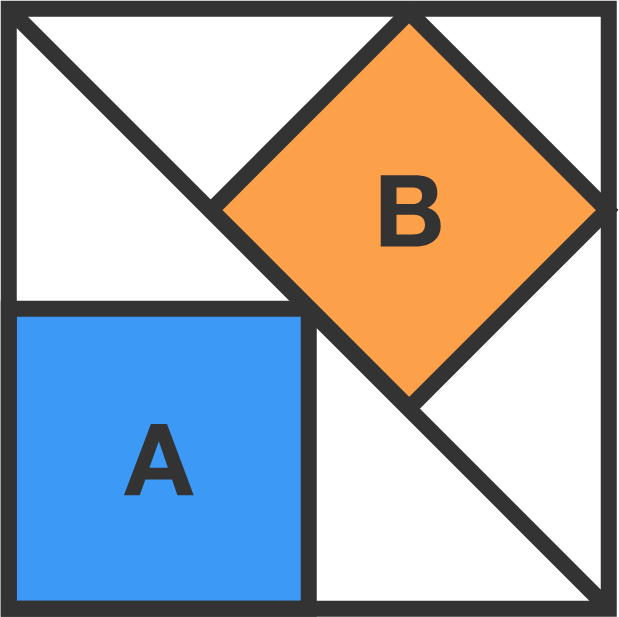# 3 squares

A diagonal divides a large, outer square into two equal parts. A smaller square is inscribed in each part. Let the area of the blue square be $A,$ and let the area of the orange square be $B.$

What is $\frac AB?$×